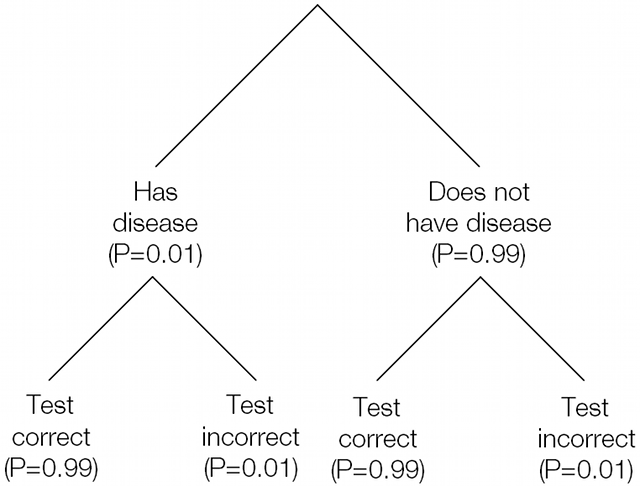# The base rate fallacy

Imagine that there is a rare genetic disease that affects 1 in every 100 people at random. There is a test for this disease that has a 99% accuracy rate: of every 100 people tested it will give the correct answer to 99 of those people.

If you have the test, and the result of the test is positive, what is the chance that you have the disease?

If you think the answer is 99% then you are incorrect; this is because of the base rate fallacy – you have failed to take the base rate (of the disease) into account.

In this situation there are four possible outcomes:

 Affected by disease Not affected by disease Test correct Affected by disease, and test gives correct result. (DC) Not affected by disease, and test gives correct result. (NC) Test incorrect Affected by disease, and test gives incorrect result. (DI) Not affected by disease, and test gives incorrect result. (NI)

This is easier to understand if we map the contents of the probability space using a tree diagram, as shown below.In two of these cases the result of the test is positive, but in only one of them do you have the disease.

P(DC) = P(Affected) × P(Test correct)
P(DC) = 0.01 × 0.99
P(DC) = 0.0099 = 1 in 101

The other case that results in a positive result, when you don’t have the disease and the test in incorrect has the same 1 in 101 probability: P(NI) = 0.0099.

Of the two remaining cases, not having the disease and getting a correct negative test result takes up the vast majority of the remaining probability space: P(NC) = 0.9801 or 1 in 1.02. The chance of having the disease and getting an incorrect test result is extremely small: P(DI) = 0.0001 or 1 in 10000.